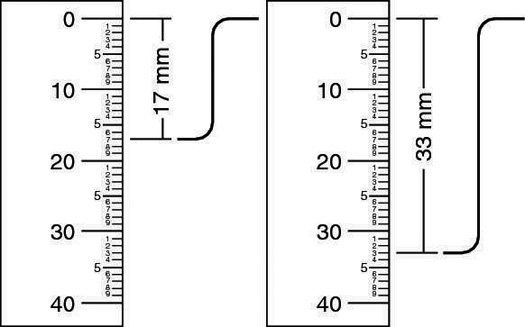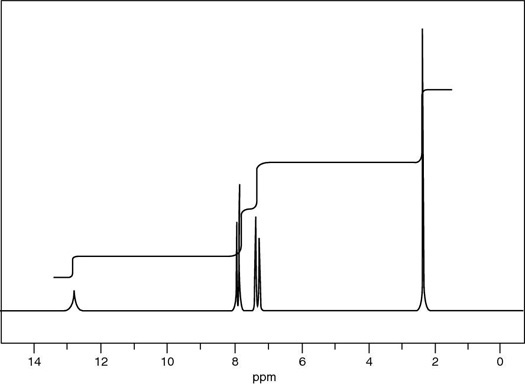##### Organic Chemistry I For Dummies, 2nd EditionWhen you use hydrogen NMR (or 1H NMR) to determine how many hydrogens are in a molecule, this only gives you the relative number of hydrogens, not the absolute number. For example, say that your relative ratio of hydrogens is 1:2, as shown here.By measuring integration curves from NMR peaks, you find a relative ratio of 1:2.
This does not necessarily mean that the peak on the left represents one hydrogen, and the peak on the right represents two hydrogens. Remember that this is the relative ratio of hydrogens, not the absolute ratio, which means that you know only that the larger peak has twice the number of hydrogens as the smaller peak; you don't know the actual number of hydrogens.

To find the absolute number of hydrogens, you must compare your ratio to the molecular formula.

If the sum of all the numbers in the relative ratio matches the number of hydrogens in the molecular formula — in this example, if there were only three hydrogens in the molecular formula — then you could say that the peak on the left represents one hydrogen and the peak on the right represents two hydrogens, because then all the hydrogens in the molecule would be accounted for.

But if the molecular formula had six hydrogens in it, you would have to multiply the relative ratio by two to make the number in your spectrum match the number in your molecular formula — so in that case the peak that integrates for one would represent two hydrogens and the peak that integrates for two would represent four hydrogens. If there were nine hydrogens, you would multiply the relative integrations by three, and so forth.8H8O2."/>

The NMR spectrum for a compound with molecular formula C8H8O2
Here's another example: Say you want to find the structure of a compound whose molecular formula is C8H8O2, and you are given its 1H NMR spectrum (shown in the figure). By measuring the heights of the integration curves, you find the relative ratio to be 1:2:2:3.

Now you need to convert that ratio into the absolute number of hydrogens that each peak represents. In this case, the molecular formula (C8H8O2) tells you that eight hydrogens are in the molecule. Because the relative ratio adds up to 8 (1 + 2 + 2 + 3), you don't have to multiply the ratio by anything; the relative ratio is also the absolute ratio. You now know that the first peak represents 1H, the second and third peaks represent 2H, and the fourth peak represents 3H.

Remember that the sum of all the hydrogens (absolute number) that you derived from the integrations must exactly match the number of hydrogens in the molecular formula. If the numbers don't match, then you need to reconsider the values of the integrals.